# PSAT Math : Percentage

## Example Questions

### Example Question #11 : How To Find The Sale Price

Molly needs to buy a new microwave. Two stores, A and B, sell the exact model microwave that Molly needs to buy; however, stores A and B are selling this microwave at two different prices. Store A is selling the microwave for its regular price of $250. Store B is having a 20% off sale on all kitchen appliances. If the regular price of the microwave at store B is$275, how much money will Molly save if she purchases the microwave at store B with the additional 20% discount compared to if she were to purchase the microwave at store A?

$25$50

$20$75

$30 Correct answer:$30

Explanation:

If Molly buys the microwave at store B at the discounted price, we need to calculate what the price of the microwave is after the 20% discount. In order to do so, we must multiply 20% with the $275 regular price of the microwave at store B. 20% is 0.20. So, 0.20 *$275 = $55 This is the discount we will receive. That means that we must subtract$55 from the regular price of $275.$275 – $55 =$220

This is the price of the microwave at store B after the 20% discount.

Now we must compare the prices from store A and B.

Store A sells the microwave for $250. Therefore,$250 – $220 =$30 saved.

### Example Question #1201 : Sat Mathematics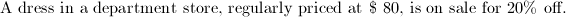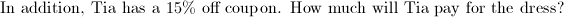Explanation:

Sale price: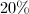off: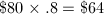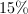coupon: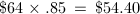Company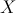is introducing its newest smartphone model, so it is reducing the price of the previous model by 85%.  If the previous model used to sell for $180, what is its new price? Possible Answers:Correct answer:Explanation: The sale price is 85% less than the original which is the same as 15% of the original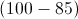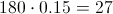### Example Question #1203 : Sat Mathematics An item is full-priced at$75. It is reduced by 10%, and then you apply a 25% off coupon to that price. There is a 7% sales tax on your purchase. How much do you pay?Explanation:

The item begins at $75. it is 10% off.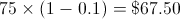You then take an additional 25% off: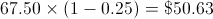There is then a 7% sales tax: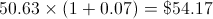### Example Question #11 : Percentage Julie goes shopping at Gap. There is a storewide sale of 30% off. She buys a sweater on clearance that gets an additional 50% off. If the sweater was originally$50, how much did she pay?

$10$17.50

$35$21.50

$25 Correct answer:$17.50

Explanation:

The original price was $50. First you take 30% off (50 * (100 - 30)/100 =$35). Then you take an additional 50% off the new price (35 * 50/100 = 17.50)

### Example Question #11 : How To Find The Sale Price

If Stacy paid $26 for a shirt at a 30%-off sale, what was the original price of the shirt? Possible Answers:Correct answer:Explanation: If the shirt is$26 after 30% was taken off, then cost of the the shirt ($26) is 70% of the original price. We set up an equation that states: 0.7x = 26 (0.7 is the decimal value of 70%) x = 26/0.7 =$37.14

### Example Question #11 : How To Find The Sale Price

A pair of shoes originally sells for $250. There is a sale, and the shoes are then sold for 20% off. The shoes are then marked down an additional 35%. If sales tax is 7%, what can you buy the pair of shoes for today, including tax? Possible Answers:$162.50

$130.00$137.00

$214.70$139.10

$139.10 Explanation: The shoes are first marked down 20%. 20% of$250 = .2 x $250 =$50

Sales price = $250 -$50 = $200 The second markdown is 35%. 35% of$200 = .35 x $200 =$70

New price = $200 -$70 = $130 Calculate the sales tax: 7% of$130 = .07 x $130 =$9.10

Total price = $130 +$9.10 = $139.10 ### Example Question #11 : Percentage A shirt at the store has a retail price of$17.50. If a customer buys the shirt and uses a 15% coupon, how much does he pay for the shirt, assuming there is no sales tax?

(Round to two decimal places.)

$15.25$15.75

$14.88$14

$14.87 Correct answer:$14.88

Explanation:

If a customer has a 15% coupon, the customer will pay 85% of the original price. To solve this problem, simply multiply the original price of the shirt by the percent of the price the customer still has to pay: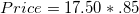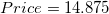Round to the nearest two decimal places, and we see that the price of the shirt is $14.88. ### Example Question #411 : Arithmetic Your friend works at a computer store. She can get a 15% discount on any item’s current price for as many items as she wants. She wants to buy a laptop for$2200 and a smartphone for $200. Local sales tax where your friend lives is 8%. What will be her total cost to buy these two items? Possible Answers:$2203.20

$2592$1848.08

$2400$2040

$2203.20 Explanation: First, find the total price of the products$2200 + $200 =$2400.

Then apply the 15% discount: $2400 * 0.15 =$360

Subtract the discount: $2400 –$360 = $2040. Then find cost of sales tax$2040 * 0.08 = 163.20

Add: $2040 + 163.20 =$2203.20.

(An alternative would be to multiply the cost by 1.08.)

### Example Question #1 : How To Find Amount Of Profit

A sunglasses kiosk at the mall makes a $50 profit for every 6 pairs of sunglasses it sells. How many pairs of sunglasses must it sell to earn$1000 profit?

20

30

300

120

100

Divide the profit per 6 pairs into the total desired profit $1000/$50 = 20.